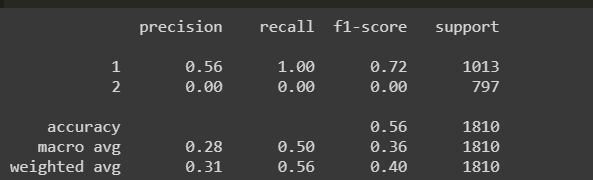# Neural Network : really high loss binary classification

Hello everybody,
I have an issues.
I had a really unbalanced datased, I rebalanced it and after I applied a Neural Network.
The problem is that it gives me high Loss and Low accuracy.
I have already applied Kfold validation, standard scaler and also testsplit.
My loss fuction is binary crossentropy because I have to do a binary classification.
Can you help me?

Could you check, if your model is still overfitting, i.e. is it predicts just a single class?
If so, could you post the model code as well as your training routine, so that we could check if for some errors?

1 Like

At beginning problem was that class were umbalced and I balanced.
Balancement works for f1 score in svc , but none improvment from f1 score of neural netowrk, it also reduce the accuracy! why? . I also tried Kfold and crossfold validation, same result. Also tried to semplify level, but nothing.
scaling:
`

X_ = dataset_train_upsampled.drop([‘LABEL’], axis=1)
y_ = dataset_train_upsampled[‘LABEL’]
X_=np.array(X_)
y_=np.array(y_)
X_train_full_, X_test_, y_train_full_, y_test_ = train_test_split(X_, y_,test_size=0.2, random_state=42)
X_train_, X_validation_, y_train_, y_validation_ = train_test_split(X_train_full_, y_train_full_, random_state=42)
scaler = StandardScaler()

X_train_ = scaler.fit_transform(X_train_)

X_validation_ = scaler.transform(X_validation_)

X_test_ = scaler.transform(X_test_)
`

The accuracy might be reduced for a balanced dataset due to the Accuracy Paradox.
If you are dealing with a highly imbalanced dataset, your model might just predict the majority class and thus get a high accuracy without “learning” anything. How does the F1 score look during training?

1 Like

F1 score is 0 for the second class, and worse for the first-one. In generally it gets worse.
I really can’t understand. I trained other models(SVC, Trees, Random forest), and f1-score improve significantly after upsampling
I have problems only with neural network. Could be possible that neural network is a model too complicated to solve this problem?
Dataset is umbalaced and training set is really poor..

just to be clear, this is the result of neural network.this one of SVC.

both after upsamplig.

Really thanks

I’m not familiar with your Keras implementation (and you might get a better answer on StackOverflow).

That being said, I’m a bit confused by the output metric for your neural network.
Your model seems to have one output neuron, which implies it should be able to predict two classes (positive and negative).
How are you creating the result table and are you sure you are passing the expected format?

never mind ptrblck! really thank you for your time and your answer